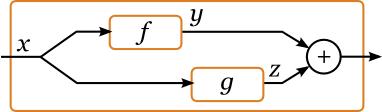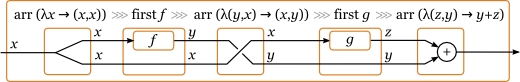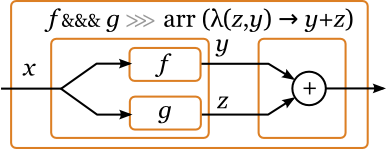# Arrows: A General Interface to Computation

Hughes's original formulation of arrows used a point-free style, which is convenient for calculating general properties, but cumbersome for specific definitions. [Pat01] proposes to extend Haskell so that arrows are almost as convenient to describe as monadic computations. The new forms are defined by translation back to standard Haskell. Here's a simple example to illustrate the notation:

```    addA :: Arrow a => a b Int -> a b Int -> a b Int
addA f g = proc x -> do
y <- f -< x
z <- g -< x
returnA -< y + z
```First, note that there are two new keywords:

proc (arrow abstraction)
is a kind of lambda, except that it constructs an arrow instead of a function.
-< (arrow application)
feeds the value of an expression into an arrow.

If you think of proc as lambda and -< as application, the above looks very much like the do-notation for monads. Bear in mind however that behind the notation lie the more general arrows, as can be seen in the Haskell translation:

```    addA f g = arr (\ x -> (x, x)) >>>
first f >>> arr (\ (y, x) -> (x, y)) >>>
first g >>> arr (\ (z, y) -> y + z)
```Of course in this particular case a much more concise translation is possible:

```    addA f g = f &&& g >>> arr (\ (y, z) -> y + z)
```but the preprocessor isn't that smart. Some more examples:

The last two examples use more fancy definitions from the experimental arrow library (see the download page).

Here are the translation rules used. (The old version was a little lighter, but less powerful.)

## Implementation

Two implementations are available:
• There is a preprocessor that reads as input a Haskell script augmented with arrow notation, and outputs a plain Haskell script. (See the download page.) It doesn't handle literate scripts, but a wrapper script is included, which runs them through the appropriate filter first.

The program is an extension of hsparser, by Sven Panne, Simon Marlow and Noel Winstanley. (The original hsparser, with bug fixes, is now in the haskell-src package of the Haskell hierarchical libraries.)

The preprocessor does no checking, so if you make an error you'll get a rather obscure message from the compiler/interpreter when it reads the output.

• The notation is also implemented directly in GHC, from version 6.2, where it is enabled by the -farrows option. Error reports for arrow notation are considerably clearer, and refer to the original source. The documentation accompanying GHC has more details.

## Bugs and Limitations

General limitations:

• Patterns that could fail aren't yet handled as specified, partly because the preprocessor currently doesn't have access to all imported modules to figure out whether constructors are unique. (And maybe the specified treatment isn't such a great idea anyway.)
• John Hughes's suggestion for top-level definitions isn't implemented yet.

The rest relate to the preprocessor, not the GHC implementation.

• Definitions in let clauses are monomorphic.
• The only Haskell extension recognized is multi-parameter type classes. Anything else needs to go in separate modules.
• The arrow combinators should be qualified in the preprocessor output, but I've left this out so I can read the output.
• The preprocessor output has been simplified, but more could be done.

Ross Paterson